Home

# Casselman’s Basis of Iwahori Vectors and the Bruhat Order

## Abstract

W. Casselman defined a basis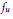${{f}_{u}}$ of Iwahori fixed vectors of a spherical representation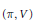$(\pi ,\,V)$ of a split semisimple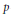$p$ -adic group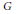$G$ over a nonarchimedean local field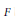$F$ by the condition that it be dual to the intertwining operators, indexed by elements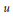$u$ of the Weyl group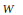$W$ . On the other hand, there is a natural basis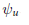${{\psi }_{u}}$ , and one seeks to find the transition matrices between the two bases. Thus, let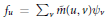${{f}_{u}}\,=\,{{\sum }_{v}}\overset{\tilde{\ }}{\mathop{m}}\,(u,\,v){{\psi }_{v}}$ and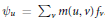${{\psi }_{u}}\,=\,{{\sum }_{v}}m(u,\,v){{f}_{v}}$ . Using the Iwahori–Hecke algebra we prove that if a combinatorial condition is satisfied, then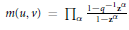$m(u,\,v)\,=\,{{\Pi }_{\alpha }}\,\frac{1-{{q}^{-1}}\,{{z}^{\alpha }}}{1-{{z}^{\alpha }}}$ , where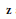$\mathbf{z}$ are the Langlands parameters for the representation and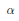$\alpha$ runs through the set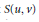$S(u,\,v)$ of positive coroots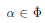$\alpha \,\in \,\hat{\Phi }$ (the dual root systemof$G$ ) such that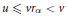$u\,\le \,v{{r}_{\alpha }}\,<\,v$ with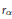${{r}_{\alpha }}$ the reflection corresponding to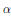$\alpha$ . The condition is conjecturally always satisfied if$G$ is simply-laced and the Kazhdan–Lusztig polynomial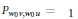${{P}_{{{w}_{0}}v,\,{{w}_{0}}u}}\,=\,1$ with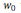${{w}_{0}}$ the long Weyl group element. There is a similar formula for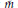$\tilde{m}$ conjecturally satisfied if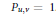${{P}_{u,\,v}}\,=\,1$ . This leads to various combinatorial conjectures.

• # Send article to Kindle

Note you can select to send to either the @free.kindle.com or @kindle.com variations. ‘@free.kindle.com’ emails are free but can only be sent to your device when it is connected to wi-fi. ‘@kindle.com’ emails can be delivered even when you are not connected to wi-fi, but note that service fees apply.

Find out more about the Kindle Personal Document Service.

Casselman’s Basis of Iwahori Vectors and the Bruhat Order
Available formats
×

# Send article to Dropbox

To send this article to your Dropbox account, please select one or more formats and confirm that you agree to abide by our usage policies. If this is the first time you use this feature, you will be asked to authorise Cambridge Core to connect with your <service> account. Find out more about sending content to Dropbox.

Casselman’s Basis of Iwahori Vectors and the Bruhat Order
Available formats
×

# Send article to Google Drive

To send this article to your Google Drive account, please select one or more formats and confirm that you agree to abide by our usage policies. If this is the first time you use this feature, you will be asked to authorise Cambridge Core to connect with your <service> account. Find out more about sending content to Google Drive.

Casselman’s Basis of Iwahori Vectors and the Bruhat Order
Available formats
×

## References

Hide All
MathJax
MathJax is a JavaScript display engine for mathematics. For more information see http://www.mathjax.org.

# Casselman’s Basis of Iwahori Vectors and the Bruhat Order

## Metrics

### Full text viewsFull text views reflects the number of PDF downloads, PDFs sent to Google Drive, Dropbox and Kindle and HTML full text views.

Total number of HTML views: 0
Total number of PDF views: 0 *Loading metrics...

### Abstract viewsAbstract views reflect the number of visits to the article landing page.

Total abstract views: 0 *Loading metrics...

* Views captured on Cambridge Core between <date>. This data will be updated every 24 hours.

Usage data cannot currently be displayed